# faceEdges

Find edges attached to specified faces

## Syntax

``EdgeID = faceEdges(g,RegionID)``
``EdgeID = faceEdges(g,RegionID,FilterType)``

## Description

example

````EdgeID = faceEdges(g,RegionID)` finds edges attached to the faces with ID numbers listed in `RegionID`.```

example

````EdgeID = faceEdges(g,RegionID,FilterType)` returns internal, external, or all edges attached to the faces with ID numbers listed in `RegionID`. This syntax is valid for 3-D geometries only.```

## Examples

collapse all

Find edges attached to the top and bottom faces of a block.

Create a block geometry.

`gm = multicuboid(3,2,1)`
```gm = DiscreteGeometry with properties: NumCells: 1 NumFaces: 6 NumEdges: 12 NumVertices: 8 Vertices: [8x3 double] ```

Plot the geometry with the face labels.

`pdegplot(gm,'FaceLabels','on','FaceAlpha',0.2)`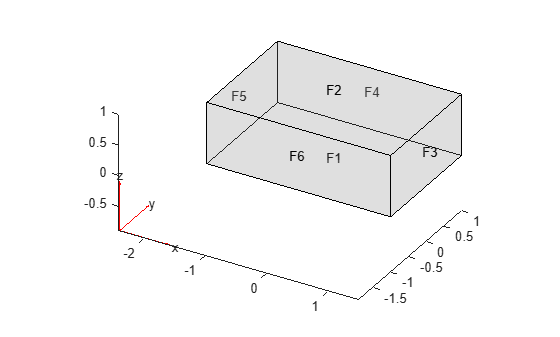Find edges attached to faces 1 and 2.

`edgeIDs = faceEdges(gm,[1 2])`
```edgeIDs = 1×8 1 2 3 4 5 6 7 8 ```

Plot the geometry with the edge labels.

```figure pdegplot(gm,'EdgeLabels','on','FaceAlpha',0.2)```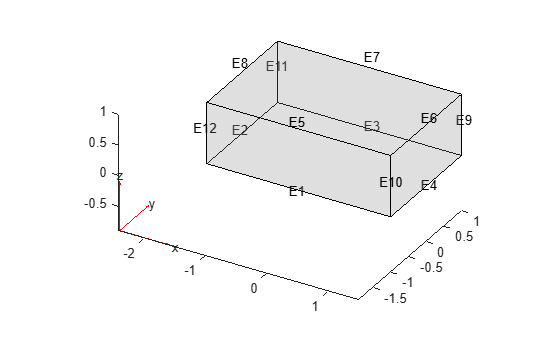Find edges attached to two faces of the L-shaped membrane.

Create a model and include this geometry. The geometry of the L-shaped membrane is described in the file `lshapeg`.

```model = createpde(); gm = geometryFromEdges(model,@lshapeg)```
```gm = AnalyticGeometry with properties: NumCells: 0 NumFaces: 3 NumEdges: 10 NumVertices: 8 Vertices: [8x2 double] ```

Plot the geometry with the face labels.

`pdegplot(gm,'FaceLabels','on')`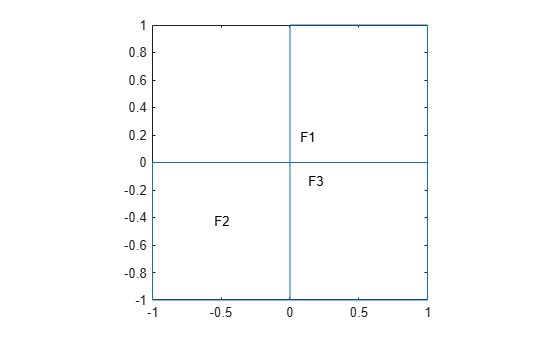Find edges attached to faces 1 and 2.

`edgeIDs = faceEdges(gm,[1 2])`
```edgeIDs = 1×8 1 2 3 6 7 8 9 10 ```

Plot the geometry with the edge labels.

```figure pdegplot(gm,'EdgeLabels','on')```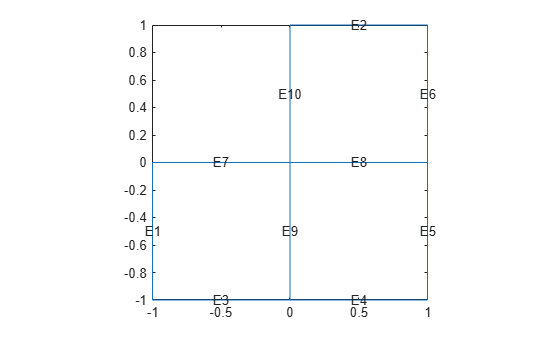Find edges attached to the side face of the inner cuboid in a geometry consisting of two nested cuboids.

Create a geometry that consists of two nested cuboids of the same height.

`gm = multicuboid([2 5],[4 10],3)`
```gm = DiscreteGeometry with properties: NumCells: 2 NumFaces: 12 NumEdges: 24 NumVertices: 16 Vertices: [16x3 double] ```

Plot the geometry with the face labels.

`pdegplot(gm,'FaceLabels','on','FaceAlpha',0.2)`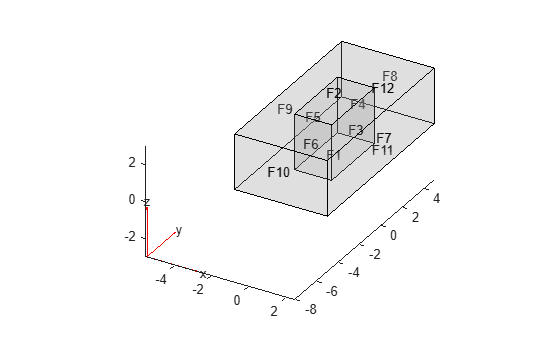Find all edges attached to the side face of the inner cuboid.

`edgeIDs = faceEdges(gm,6)`
```edgeIDs = 1×4 1 5 10 12 ```

From all edges attached to that face, return the edges attached to only the internal faces. Internal faces are faces shared between multiple cells.

`edgeIDs = faceEdges(gm,6,'internal')`
```edgeIDs = 1×2 10 12 ```

From all edges attached to that face, return the edges attached to the external faces.

`edgeIDs = faceEdges(gm,6,'external')`
```edgeIDs = 1×2 1 5 ```

Plot the geometry with the edge labels.

`pdegplot(gm,'EdgeLabels','on','FaceAlpha',0.2)`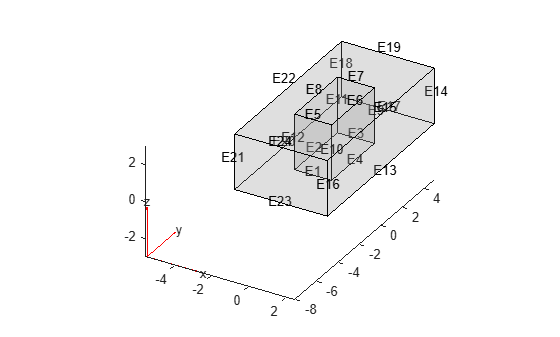## Input Arguments

collapse all

Geometry, specified as a `DiscreteGeometry` or `AnalyticGeometry` object.

Face ID, specified as a positive number or a vector of positive numbers. Each number represents a face ID.

Type of edges to return, specified as `'internal'`, `'external'`, or `'all'`. Depending on this argument, `faceEdges` returns these types of faces for a 3-D geometry:

• `'internal'` — Edges attached to only internal faces. Internal faces are faces shared between multiple cells.

• `'external'` — Edges attached to only external faces. External faces are faces not shared between multiple cells.

• `'all'` — All edges attached to the specified cells.

## Output Arguments

collapse all

IDs of edges attached to the specified faces, returned as a positive number or a vector of positive numbers.

## Version History

Introduced in R2021a